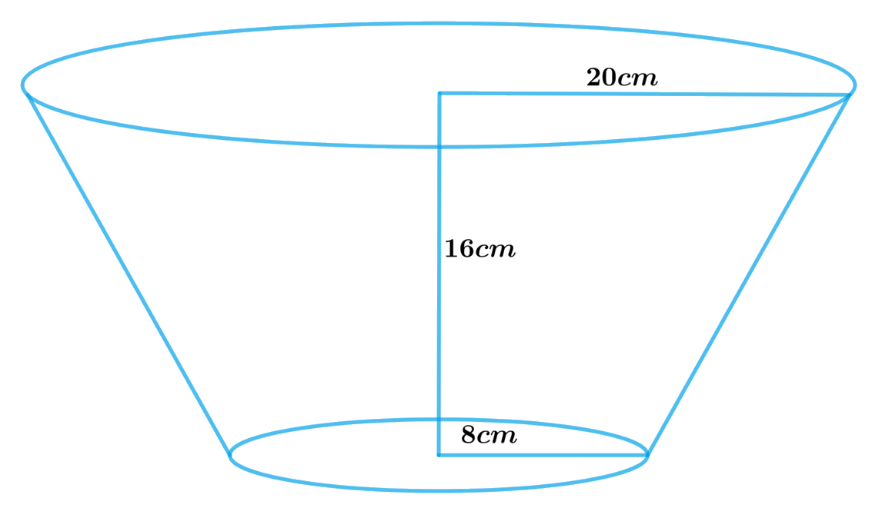# Ex.13.4 Q4 Surface Areas and Volumes Solution - NCERT Maths Class 10

Go back to  'Ex.13.4'

## Question

A container, opened from the top and made up of a metal sheet, is in the form of a frustum of a cone of height $$16 \,\rm{cm}$$ with radii of its lower and upper ends as $$8 \,\rm{cm}$$ and $$20 \,\rm{cm}$$, respectively. Find the cost of the milk which can completely fill the container, at the rate of  $$₹\,20$$ per liter. Also find the cost of metal sheet used to make the container, if it costs  $$₹\,8$$ per $$100 \rm \,cm^2.$$

$$(\rm{Take}\, \pi = 3.14)$$

Video Solution
Surface Areas And Volumes
Ex 13.4 | Question 4

## Text Solution

What is Known?

A container opened from the top and made up of a metal sheet is in the shape of a frustum of a cone with of height $$16\rm{ cm}$$, radius of lower end $$8\rm{ cm}$$ and radius of upper end $$20 \rm{cm.}$$

Cost of $$1$$ litre of milk is $$₹ 20$$ and Cost of $$100\,\rm{cm^2}$$ of metal sheet is $$₹ 8.$$

What is Unknown?

Cost of the milk which can completely fill the container and Cost of metal sheet used to make the container

Reasoning:To find the cost of milk:

Since the container is in the shape of a frustum of a cone, so volume of milk which can completely fill the container will be equal to volume of frustum of cone.

Therefore, volume of milk $$=$$ volume of frustum of cone

We will find the volume of the frustum by using formula;

Volume of frustum of a cone\begin{align} = \frac{1}{3}\pi h\left( {r_1^2 + r_2^2 + {r_1}{r_2}} \right)\end{align}

where $$r1, r2$$ and $$h$$ are the radii and height of the frustum of the cone respectively.

Once we know the volume of milk, we need to convert it into litre as the cost of milk is in terms of $$₹$$ per litre.

\begin{align}1{\text{ }}litre &= 1000c{m^3}\\1c{m^3} &= \frac{1}{{1000}}\text{litre}\end{align}

To find the cost of metal sheet:

Since the container is open from the top the metal sheet will only contain CSA of frustum of the cone and area of the lower circular end.

Therefore,

Area of metal sheet required to make the container $$=$$ CSA of frustum of the cone $$+$$ Area of lower circular end

We will find the CSA of the frustum by using formulae;

CSA of frustum of a cone$$= \pi \left( {{r_1} + {r_2}} \right)l$$

Slant height\begin{align}l = \sqrt {{{\left( {{r_1} - {r_2}} \right)}^2} + {h^2}} \end{align} $$(r1 > r2)$$

where $$r1, r2,h$$ and $$l$$ are the radii height and slant height of the frustum of the cone respectively.

Area of the lower circular end $$= \pi r_2^2$$

where $$r2$$ is the radius of the lower circular end.

Steps:

Height of the frustum of cone, $$h =16 \,\rm{cm}$$

Radius of the upper end, $${r_1}=20 \,\rm{cm}$$

Radius of the lower end, $${r_2}=8\;\rm{cm}$$

Slant height of the frustum,

\begin{align}l &= \sqrt {{{\left( {{r_1} - {r_2}} \right)}^2} + {h^2}}\\l &= \sqrt {{{\left( {20cm - 8cm} \right)}^2} + {{\left( {16cm} \right)}^2}} \\&= \sqrt {144c{m^2} + 256c{m^2}} \\&= \sqrt {400c{m^2}} \\&= 20cm\end{align}

Volume of milk which can completely fill the container $$=$$ Volume of the frustum of Cone

\begin{align}&= \frac{1}{3}\pi h\left( {r_1^2 + r_2^2 + {r_1}{r_2}} \right)\\&= \frac{1}{3} \times 3.14 \times 16cm \times \left( {{{\left( {20cm} \right)}^2} + {{\left( {8cm} \right)}^2} + 20cm \times 8cm} \right)\\&= \frac{1}{3} \times 3.14 \times 16cm \times \left( {400c{m^2} + 64c{m^2} + 160c{m^2}} \right)\\&= \frac{1}{3} \times 3.14 \times 16cm \times 624c{m^2}\\&= 10449.92c{m^3}\\&= \frac{{10449.92}}{{1000}}\,\text{litre}\\&= 10.44992\,\text{litre}\\&= 10.45\,\text{litre}\end{align}

Cost of $$1$$ lite of milk \begin{align}=₹ \;20\end{align}

Therefore, cost of $$10.45$$ liter of milk \begin{align} = 20 \times 10.45 = \,₹\, 209 \end{align}

Area of metal sheet required to make the container $$=$$ CSA of frustum of the cone $$+$$ Area of lower circular end

\begin{align} & =\pi \left( {{r}_{1}}+{{r}_{2}} \right)l+\pi r_{2}^{2} \\ & =\pi \left[ \left( {{r}_{1}}+{{r}_{2}} \right)l+r_{2}^{2} \right] \\ & =3.14\times \left[ \begin{array} & \left( 20cm+8cm \right)\times \\ 20cm+{{\left( 8cm \right)}^{2}} \\ \end{array} \right] \\ & =3.14\times \left[ 560c{{m}^{2}}+64c{{m}^{2}} \right] \\ & =3.14\times 624c{{m}^{2}} \\ & =1959.36c{{m}^{2}} \\ \end{align}

Cost of $$100 \rm cm^2$$ of metal sheet $$= \,₹\;8$$

Therefore, cost of $$1959.36c{m^2}$$ of metal sheet \begin{align}= ₹ \frac{8}{{100}} \times 1959.36\end{align}

\begin{align}&= ₹ 156.7488\\ &= ₹ 156.75\end{align}

Cost of milk which can completely fill the container is $$₹\;209$$

Cost of metal sheet required to make the container is $$₹\; 156.75$$

Learn from the best math teachers and top your exams

• Live one on one classroom and doubt clearing
• Practice worksheets in and after class for conceptual clarity
• Personalized curriculum to keep up with school